# electromagnetic

Explain how the attenuation constant value is an important factor for propagation of a transverse electromagnetic wave in a medium. (5 marks)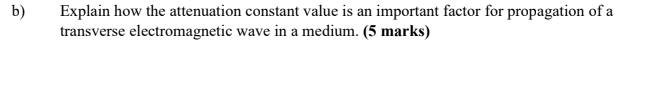This Homework Help Question: "electromagnetic" No answers yet.

We need 9 more requests to produce the answer to this homework help question. Share with your friends to get the answer faster!

1 /10 have requested the answer to this homework help question.

Once 10 people have made a request, the answer to this question will be available in 1-2 days.
All students who have requested the answer will be notified once they are available.

#### Earn Coin

Coins can be redeemed for fabulous gifts.

Similar Homework Help Questions
• ### In a medium characterised by a = 0,4 = Ho,€, an electromagnetic wave is described by;...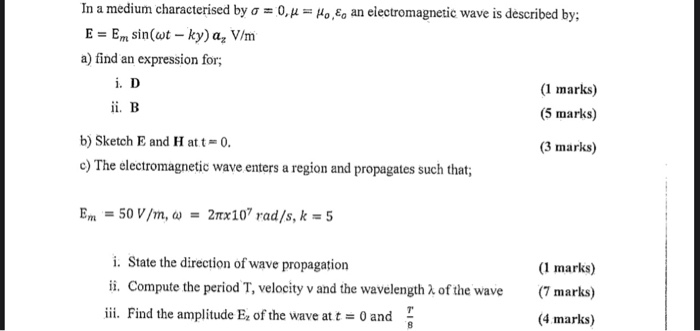In a medium characterised by a = 0,4 = Ho,€, an electromagnetic wave is described by; E = Em sin(wt -ky) a, V/m a) find an expression for; (1 marks) ii. B (5 marks) b) Sketch E and Hatt=0. (3 marks) c) The electromagnetic wave enters a region and propagates such that; i. D Em = 50 V /m, do = 2mx10? rad/s, k = 5 i. State the direction of wave propagation ii. Compute the period T, velocity v...

• ### Electromagnetic Field : wave propagation !

1 ) A satellite in Geosynchronous orbit (37000 Km above the equator ) is used for communication at 30 GHz . The Atmosphere is 15 Km thick . Assume free space abovethe atmosphere and plane wave propagation . Properties of the atmosphere are ε= 1.05 ε0 , μ = μ0 and σ=10-6 S/m . calculate :a) the phase velocity in air and in free space .b) The attenuation and phase constant in air and in free space .c) the propagation...

• ### (20 Marks) 5.,) A wave is transmitted through an antenna with a frequency of 100 MHE,...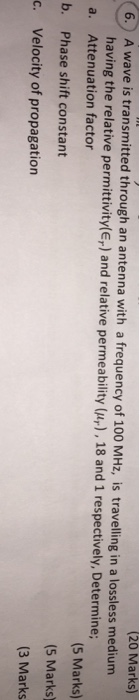(20 Marks) 5.,) A wave is transmitted through an antenna with a frequency of 100 MHE, is travelling in a lossle having the relative permittivity(E,) and relative permeability (ur), 18 and 1 respectively, Determine; a. Attenuation factor b. Phase shift constant c. Velocity of propagation (5 Marks) (5 Marks) (3 Marks

• ### 6.) A wave is transmitted through an antenna with a frequency of 100 MHz, is travelling...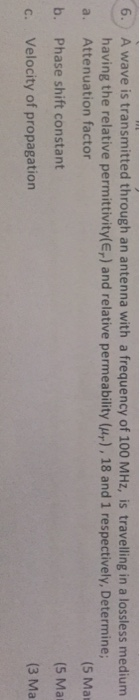6.) A wave is transmitted through an antenna with a frequency of 100 MHz, is travelling in a lossless medium (5 Ma (5 Mar (3 Ma having the relative permittivity(Er) and relative permeability (),18 and 1 respectively, Determine; a. Attenuation factor b. Phase shift constant c. Velocity of propagation

• ### 2A uniform electromagnetic plane wave is normally incident norm the air region (medium #1 with intrinsic medium (medium #2 with intrinsic k,- and wavenumber k ) upon the planar interface separati...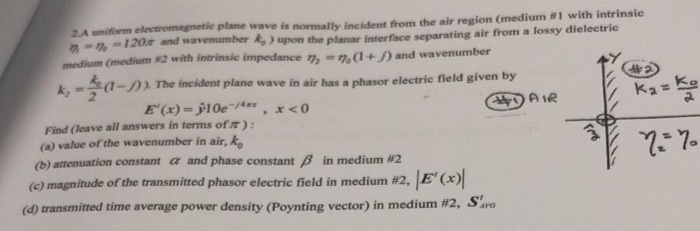2A uniform electromagnetic plane wave is normally incident norm the air region (medium #1 with intrinsic medium (medium #2 with intrinsic k,- and wavenumber k ) upon the planar interface separating air from a lossy dielectric impedance 'h-%(I + J) and wavenumber (1-)).The incident plane wave in air has a phasor electric field given by 2. Find (leave all answers in terms ofm: (a) value of the wavenumber in air, ko (b) attenuation constant α and phase constant β (c)...

• ### 5. In a lossless nonmagnetic medium with er = 4, E(y,t) = 20 cos(108nt +By+)a, V/m...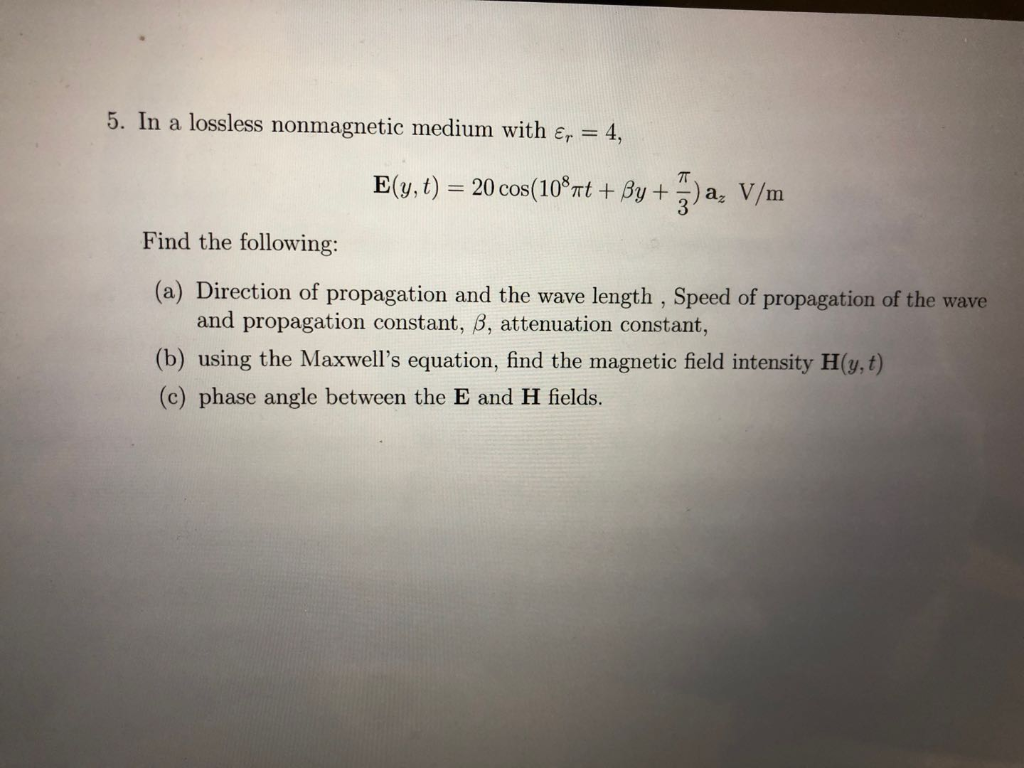5. In a lossless nonmagnetic medium with er = 4, E(y,t) = 20 cos(108nt +By+)a, V/m Find the following: (a) Direction of propagation and the wave length , Speed of propagation of the wave and propagation constant, B, attenuation constant, (b) using the Maxwell's equation, find the magnetic field intensity H(y,t) (c) phase angle between the E and H fields.

• ### Problem 6. Electromagnetic plane waves in a lossy medium The electric field of an electromagnetic plane wave traveling in a lossy medium can be written as where z is the distance, t is time, and f...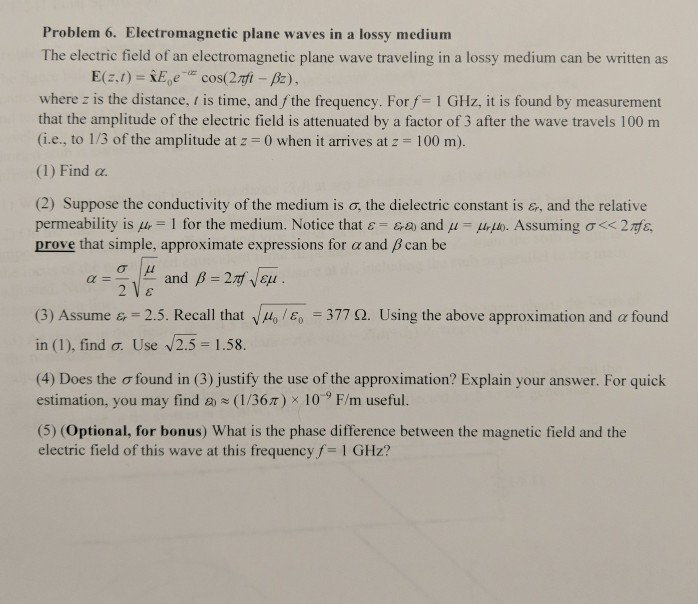Problem 6. Electromagnetic plane waves in a lossy medium The electric field of an electromagnetic plane wave traveling in a lossy medium can be written as where z is the distance, t is time, and fthe frequency. For f 1 GHz, it is found by measurement that the amplitude of the electric field is attenuated by a factor of 3 after the wave travels 100 m (i.e., to 1/3 of the amplitude at z-0 when it arrives at z- 100...

• ### Electromagnetic plane waves in a lossy medium field of an electromagnetic plane wave traveling in a lossy medium can be written as 6. The where z is the distance, t is time, and f the frequency....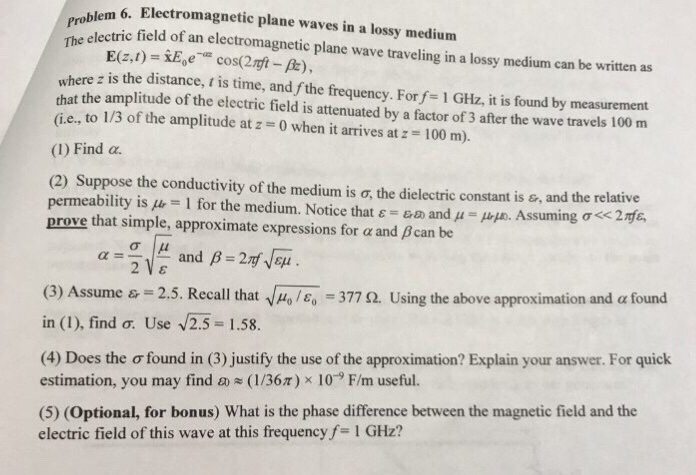Electromagnetic plane waves in a lossy medium field of an electromagnetic plane wave traveling in a lossy medium can be written as 6. The where z is the distance, t is time, and f the frequency. For f 1 GHz, it is found by measurement that the amplitude of the electric field is attenuated by a factor of 3 after the wave travels 100 m (ie, to 130f the amplitude at z = 0 when it arrives at z-100 m)....

• ### blem 6. Electromagnetic plane waves in a lossy medium tric field of an electromagnetic plane wave...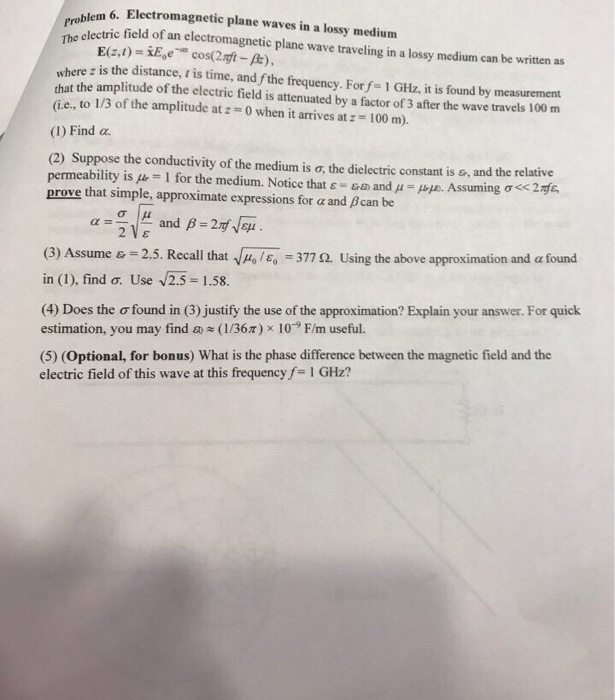blem 6. Electromagnetic plane waves in a lossy medium tric field of an electromagnetic plane wave traveling in a lossy medium can be written as The where z is the distance, t is time, and fthe frequency. Forf- 1 GHz, it is found by measurement that the amplitude of the electric field is attenuated by a factor of 3 after the wave travels 100 m (ie, to 1/3 of the amplitude at: 0 when it arrives at : -100 m)....

• ### 2. (a) Consider the propagation of a plane electromagnetic wave in a conductor. Show that the wav...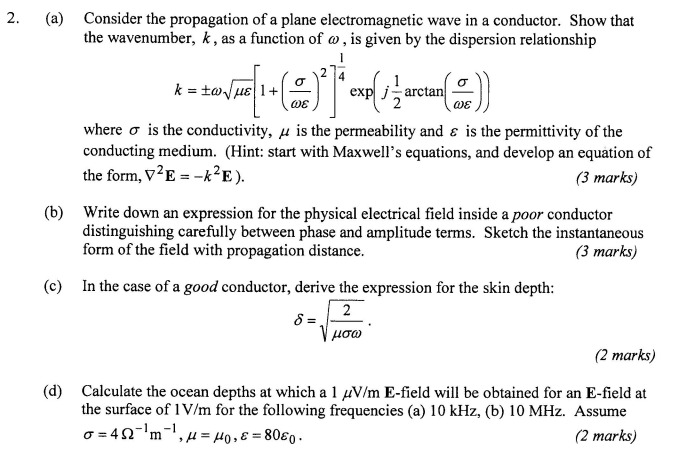2. (a) Consider the propagation of a plane electromagnetic wave in a conductor. Show that the wavenumber, k, as a function of a, is given by the dispersion relationship ex ωε ωε where σ is the conductivity, μ is the permeability and ε is the permittivity of the conducting medium. (Hint: start with Maxwell's equations, and develop an equation of the form, V" E -k 2 E ). (3 marks) (b) Write down an expression for the physical electrical field...